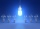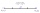# Examples for 8th grade - page 117

1. Calories 2Ben eats approximately 2400 calories per day. His wife Sarah eats 5/8 as much. How many calories does Sarah eat per day?
2. MisterMister ignacio paid Php.13693.75 for a television set that originally cost Php.15,650 . Find the rate of discount. Please show your solution please please please.
3. Midpoint 5FM=3x-4, MG=5x-26, FG=? Point M is the midpoint of FG. Use the given information to find the missing measure or value.
4. Sum of dividendIf X is divided separately 2.63 and 1.40. And the results of the individual divisions are added to give a result less than X. What is X?
5. Exchange ratesIf the Canadian dollar appreciated by C\$0.005 relative to the US dollar, what would be the new value of the Canadian dollar per US dollar? Assume the current exchange rate was US\$1 = C\$0.907.
6. Midpoint 6FM=8a+1, FG=42, a=? Point M is the midpoint of FG. Find unknown a.
7. PersonsPersons surveyed:100 with result: Volleyball=15% Baseball=9% Sepak Takraw=8% Pingpong=8% Basketball=60% Find the average how many like Basketball and Volleyball. Please show your solution.
8. Find midpointFM=5y+13, MG=5-3y, FG=? M is the midpoint of FG. Use the given information to find the missing measure or value.
9. Days2minutesWrite a formula that converts x days to y minutes. The formula is:

We apologize, but in this category are not a lot of examples.
Do you have an interesting mathematical example that you can't solve it? Enter it, and we can try to solve it.

To this e-mail address, we will reply solution; solved examples are also published here. Please enter e-mail correctly and check whether you don't have a full mailbox.